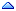Home » SQL & PL/SQL » SQL & PL/SQL » Group additions with respect to number systems
Group additions with respect to number systems Thu, 10 January 2002 18:37C.A.V.B.Srinivas Messages: 1Registered: January 2002 Junior Member
Hi,

The ordinary group additions in oracle is w.r.t
10 (decimal addtion). If I want to add w.r.to the passes parameter ( say 24 or 20,8). How does this addtion be handled in SQL.

Ex..
If There are two quantities say qty=20, qty= 26
then the below query will return me a result of 46 but
my addition W.R.T 24 is 1.22 . To make this addition how can I pass parameter 24 to the built in sum method
or some User defined Group function.
Re: Group additions with respect to number systems [message #37035 is a reply to message #37026] Fri, 11 January 2002 02:31pratap kumar tripathy Messages: 660Registered: January 2002 Senior Member
Hi,

there are several way:

1. parameterize cursor:
cursor xyz(p_test number) is
select sum(qty)
from table
where col=ptest;
2. create a function and do the above
3.in plsql
select sum(qty) into v_var
from table
where col=ptest;

cheers
pratap
Re: Group additions with respect to number systems [message #37066 is a reply to message #37026] Mon, 14 January 2002 17:57Prataap Messages: 4Registered: January 2002 Junior Member
Thanks Prataap ,
But one thing, I want the sum of 5 numbers in octal number system. For Example

7,10,20,9,12 are the numbers in decimal number system. Now if I convert it to Octal number system
then I will get 7,12,24,11,14.
Now I want the sum of 7,12,24,11,14 . do we have direct function something like sum(colname,radix) so that I can get the sum directly.
Re: Group additions with respect to number systems [message #37068 is a reply to message #37026] Mon, 14 January 2002 19:21Suresh Vemulapalli Messages: 624Registered: August 2000 Senior Member
there is no direct function to get that result.

you can try this:

this works for octal system

select sum(col1),sum(to_number(substr(dump(col1,8),instr(dump(col1,8),',')+1))-1) "octal_sum" from table_name;

col1 is number column.
 Previous Topic: SQLLDR, HOW TO IMPORT MULTI-LINES DATA? Next Topic: dELETE
Goto Forum:

Current Time: Sat Jun 10 10:09:24 CDT 2023